# Ratio of counts

There are 15 boys and 13 girls in the class. What are the ratio of boys and girls?

r =  15:13

### Step-by-step explanation:Did you find an error or inaccuracy? Feel free to write us. Thank you!Tips to related online calculators
Need help to calculate sum, simplify or multiply fractions? Try our fraction calculator.
Check out our ratio calculator.

## Related math problems and questions:

• The ratioThe ratio of girls to boys in Mrs. White's class is 3:2.  If there are 12 boys, how many girls are in the class room?
• Boys and girls 8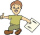In a class of 35 boys and girls that has a ratio of 2:5, 5 boys are absent and 5 boys are present. How many students are present?
• PupilsThere are 32 pupils in the classroom, and girls are two-thirds more than boys. a) How many percents are more girls than boys? Round the result to a whole percentage. b) How many boys are in the class? c) Find the ratio of boys and girls in the class. Writ
• A class IV.AIn a class there are 15 girls and 30 boys.  What fraction of the class represents the boys?
• A class IV.CIn a class 2/5 were boys. 30 were girls. How many more girls than boys are there?
• GirlsThe boys and girls in the class formed groups without the rest of the fives, two girls and three boys. There are six girls missing to create mixed pairs (1 boy and one girl). How many girls are in the classroom?
• Boys to girlsThe ratio of boys to girls at a party is 3:5. If six more boys arrived and four girls left the party, the ratio of boys to girls would be 5:6. How many people were at the party initially?
• Ski class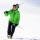Class attend 30 boys and some number of girls. Ski training was attended by 28 boys and all the girls, which was 95% of all students. How many girls attend this class? How many percent is that?
• Prospering studentsThere are girls and 30 boys in the class. Boys prospering 28, girls all. How many girls are in the class and how many boys are there, if all the prospering students are 95%?
• Boys and girls 2The ratio of boys to girls in math club is 4:3 . After 8 more girls joined the Club, the ratio become 1:1. How many members are there in the club now?
• Two prizes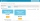In a class of 15 pupils (7 boys and 8 girls), Teacher Mae is giving away a random prize. What is probability that both prizes are won by girls?
• ClassIn a class are 32 pupils. Of these are 8 boys. What percentage of girls are in the class?
• Competition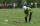15 boys and 10 girls are in the class. On school competition of them is selected 6-member team composed of 4 boys and 2 girls. How many ways can we select students?
• The classroom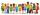In the seventh class the number of boys and girls are at a ratio of 4:5. Boys are 16. How many total students are in the classroom?
• ClassroomThere are eighty more girls in the class than boys. Boys are 40 percent and girls are 60 percent. How many are boys and how many girls?
• FortyForty five of the 80 students were girls. What is the ratio of girls to boys?
• Boys and girlsThere are eight boys and nine girls in the class. There were six children on the trip from this class. What is the probability that left a) only boys b) just two boys